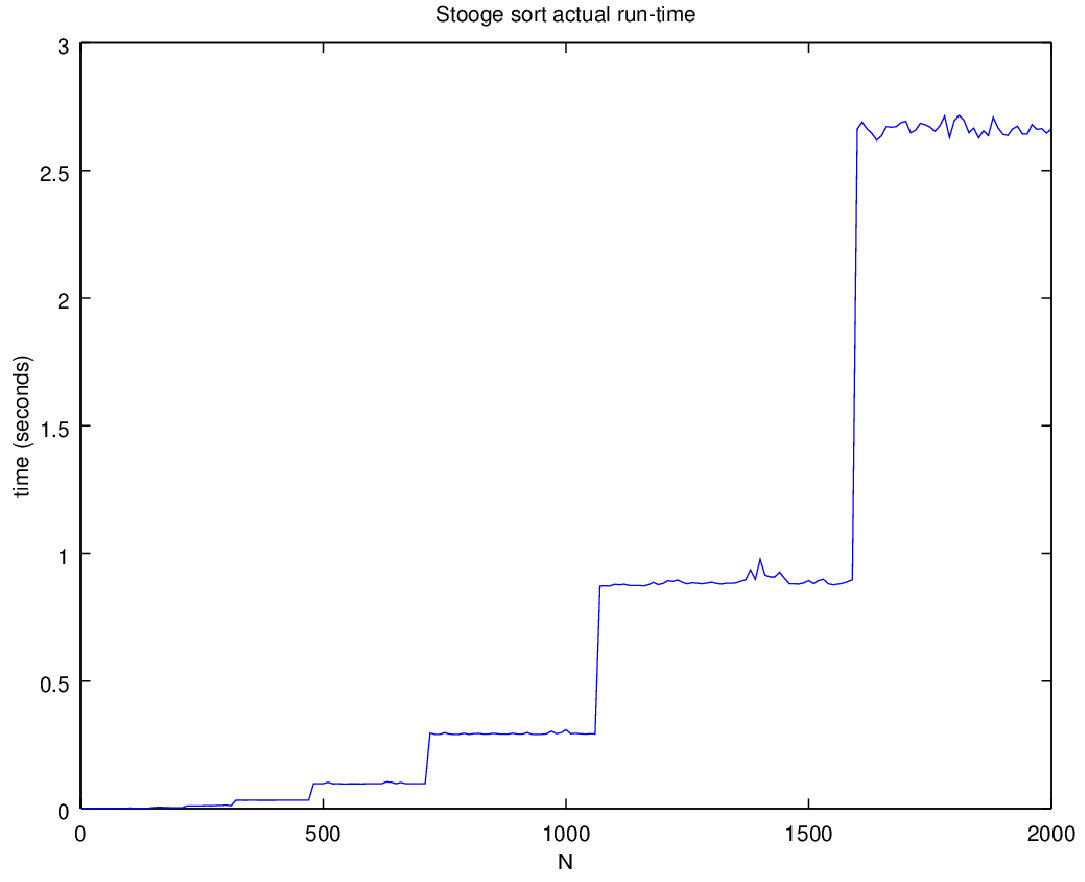# Stooge Sort

May 2018 · 4 minute read

In this post, we’ll have a look at Stooge sort, which is a recursive sorting algorithm with a peculiar time complexity. We’ll start by giving a description of the algorithm and use induction to prove that it works. Then, we’ll prove the time complexity using the Master Theorem and give a short explanation why we get such a weird plot for the time/input size.

### Algorithm

The way Stooge sort works is like this:

1. IF the first and last element in the list are not sorted, swap them around
2. IF we have more than 2 element in our list:
• stooge sort the first 2/3rds of the list
• stooge sort the last 2/3rds of the list
• stooge sort the first 2/3rds of the list, again!

### Code

void stoogeSort(int v[], int left, int right) {
if (v[left] > v[right])
swap(&v[left], &v[right]);
if (right - left + 1 >= 3) {
// Floor of the delimitator.
int delimitator = (right - left + 1) / 3;
stoogeSort(v, left, right - delimitator);
stoogeSort(v, left + delimitator, right);
stoogeSort(v, left, right - delimitator);
}
}


### Proof

Let’s use strong induction to prove that the algorithm works.

Let P(N): stooge_sort returns a sorted array for all values $$\leq$$ N, $$\forall$$ a N natural number.

#### I. Base case(2):

Obviously, the algorithm works for arrays of size 2 or smaller.

#### II. Induction step:

Suppose that stooge_sort works for all arrays of size = k or smaller. Knowing that, we must prove that it also works for arrays of size = k + 1 or smaller. Now let’s take an array A and two indexes pointing to the beginning and the end of the array, i and j, respectively, such that j - i + 1 = k + 1. Let delimitator = $$\left\lfloor\frac{k + 1}{3}\right\rfloor$$ because we need the floor of $$\frac{size}{3}$$. Now, we have that:

1) stooge_sort(A, i, j - delimitator) returns A with the first two thirds sorted.

2) stooge_sort(A, i + delimitator, j) returns A with the last 2 thirds sorted. At this stage, the greatest elements are in the last third. We must sort the first 2 thirds.

3) stooge_sort(A, i, j - delimitator) returns A with all the elements sorted.

This works because we know that any array of size smaller or equal to k can be sorted using stooge_sort $$\Rightarrow P(k + 1)$$: stooge_sort returns a sorted array for all values $$\leq$$ k + 1

#### III. Conclusion step:

From (I) and (II), it results that $$P(N)$$ is true $$\forall$$ N a natural number.

### Time complexity

We have proven the correctness of the algorithm, so now we should calculate its asymptotic time complexity. We shall use the Master Theorem for this step. The recurrence is $$T(n) = 3T(\frac{2n}{3}) + O(1)$$ with parameters:

• a = 3;
• b = $$\frac{3}{2}$$;
• c = 0;

Clearly, $$c < log_b{a}$$. Therefore, $$T(n) \in \Theta( n^{log_{\frac{3}{2}}3} ) = \Theta(n^{2.7095...})$$. It has a polynomial asymptotic complexity, but we can see that it is worse than quicksort, mergesort or even bubblesort!

### Graph

Let’s take a look at the graph.We would expect that the actual time complexity have a smooth, polynomial-like curvature; however, when we compute the time spent on each input size, we might be surprised to see that it has a staircase-like growth. It is relatively constant for some values of n, then it suddenly increases by $$\sim log_{\frac{3}{2}}3 \approx 2.71$$, repeating this pattern ad infinitum. One can clearly see this in the graph, where the values jumps from $$\sim1$$ sec to $$\sim2.7$$ sec.

The first few values of the input right before the time increases are: 2, 3, 4, 6, 9, 13, 19… That is a geometric progression with a ratio of $$\frac{3}{2}$$, which is, indeed, the base of the logarithm! This growth can be explained by the fact that at level k, the depth of the recursion tree is $$k = log_{\frac{3}{2}}n$$, because:

At level k, the size of the array is $$(\frac{2}{3})^k\times n$$. At the leaves, it is 1, so $$(\frac{2}{3})^k\times n = 1$$. By applying the rules of logs we iteratively get:

$$klog_{\frac{2}{3}}\frac{2}{3} = log_{\frac{2}{3}}\frac{1}{n}$$

$$k = -log_{\frac{2}{3}}n$$

$$k = log_{\frac{3}{2}}n$$

Q.E.D.